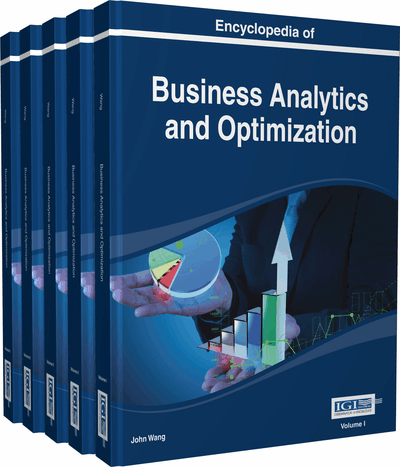# High-Dimensional Statistical and Data Mining Techniques

Gokmen Zararsiz (Hacettepe University Ankara, Turkey)
DOI: 10.4018/978-1-4666-5202-6.ch102

Top

## Background

HDD refers to data whose number of dimension is at least larger than the dimensions considered in classical multivariate analysis in statistical theory. In many fields, it is probable to work with data in which the number of variables is more than the number of observations. Richard Bellman mentioned the difficulties of function optimization by exhaustive enumeration in the function domain and referred to the problem as the “curse of dimensionality” (Bellman, 1961). If we consider 10 data points simulated from uniform distribution, then let d indicate the number of dimensions. The data points look agglomerated with each other in one dimension (d=1). The data look more dispersed in two dimensions (d=2) and even more so in three dimensions (d=3). In a statistical problem, 10d evaluations of a function are needed and this may involve huge computational cost, even for a reasonable value of d (Verleysen, 2003).

This curse of dimensionality makes classical statistical algorithms inapplicable due to their shortcomings. For instance, linear discriminant analysis is one of the powerful statistical method for predicting categorical outcomes. However, this method is very flexible in the presence of high number of correlated variables and overfits in classifying this kind of data. Conversely, it is very rigid and underfits the data when the class boundaries are nonlinear and complex (Hastie, Buja, & Tibshirani, 1995). These problems are arised from the covariance structure of the data and unpredictability of this structure makes this method inapplicable to HDD. Most of the multivariate statistical methods have similar problems based on the covariance structure of data. Also, meeting the assumptions of multivariate statistical techniques in HDD, such as multivariate normality and homogeneity of covariance matrices is troublesome.

Many techniques have been proposed as a modified version of the classical techniques to analyze HDD. For instance, the S test (Tusher, Tibshirani, & Chu, 2001) is a modified form of t test for class comparison analysis of HDD due to the difficulty of error variance estimation. The penalized logistic regression technique (Zhu & Hastie, 2004) is a modified version of the ordinary logistic regression technique for HDD classification. A similar relation exists between penalized discriminant analysis (Hastie, Buja, & Tibshirani, 1995) and Fisher’s linear discriminant analysis.

## Key Terms in this Chapter

Market Basket Data Analysis: Market basket analysis aim to find the purchasing habits of customers by examining the product combinations of their baskets.

Multiple Testing Adjustment: An approach to control the type-I error, when large number of comparisons are applied.

Overfitting/Underfitting: Overfitting occurs when excellent performance is seen in training data, but poor performance is seen in test data. Underfitting occurs when the model is too simple in which poor performance is seen in both training and test data.

Poisson Distribution: The discrete distribution which is used to model the number of events existing in a given time interval.

Uniform Distribution: A probability distribution which can be either discrete or continuous and all outcomes are equally likely.

Multivariate Normality: Multivariate normality is an assumption in multivariate statistics. In this assumption, continuous variables should follow a multivariate normal distribution to apply related analysis. Multivariate normality can be tested in MVN package of R ( Korkmaz, 2013 ).

Prototype Based Clustering: A type of clustering in which each observation is assigned to its nearest prototype (centroid, medoid, etc.).

Supervised-Unsupervised Learning: Supervised and unsupervised learning is the development of data mining models to extract information with and without using class variable, respectively. In most data mining applications, supervised learning refers to classification and regression analysis, while unsupervised learning refers to clustering analysis.

DNA Microarray: A DNA microarray is the collection of microscopic DNA spots fixed to a solid surface.

Homogeneity of Covariance Matrices: Covariance matrix is the matrix whose element in the a ij position is the covariance between the i th and j th elements of a random vector and the diagonal elements are the individual variances. Many multivariate statistical methods are applicaple based on the assumption of equality/homogeneity of covariance matrices if different groups.

## Complete Chapter List

Search this Book:
Reset